Hello Guest it is January 23, 2022, 09:31:54 AM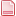Author Topic: DRO Code differences  (Read 11828 times)

0 Members and 1 Guest are viewing this topic.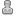smurph•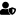•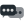1,475
• "That there... that's an RV."Re: DRO Code differences
« Reply #10 on: April 27, 2014, 08:19:13 PM »
The net does not have our API calls.  The documentation is in progress but it is a LOT of slow tedious work.

Here is a quick look at the screen API call available to LUA inside the Mach4GUI:

(All functions return a result code as their first return value.  0 == success)

number scr.ShowPage(string pageName); -- Show a screen page by name.
number, string scr.GetProperty(string ctrlName, string propName); -- Get a control's property value.
number scr.SetProperty(string NctrlName, string propame, string value); -- Set a control's property value.
number scr.ExecMdi(string ctrlName); -- Execute the contents of a named MDI control.
number, number scr.GetCurrentPage(); -- Get the current page.
number, number scr.IsControl(string ctrlName); -- Is this a valid control?
number, number scr.IsProperty(string ctrlName, string propName); -- Is this a valid control and property combination?
number scr.ButtonClick(string ctrlName); -- Simulate a button click event.
number scr.ButtonUp(string ctrlName); -- Simulate a button up event.
number scr.ButtonDown(string ctrlName); -- Simulate a button down event.

A word of warning...  DO NOT use loops in the PLC script.  It is a script that is designed to be executed like a PLC; top down.  The frequency of the script can be set in the screen set properties.  There is a lot of power here.  But with power comes great responsibility because it has the ability to completely ruin your day if you are careless!  There is nothing preventing you from adding loops or setting the PLC script to run every 1 millisecond.  It will happily let you do these things with a smile.  So be careful!

SteveYa-Nvr-No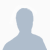•992Re: DRO Code differences
« Reply #11 on: April 27, 2014, 09:27:04 PM »
Thanks Steve

How would you use a function call from the PLC script, thought that would be better for computing the angles and distances. But fought that so guess I learned the hard way about the loops.

getAngle(Yval,Zval);
scr.SetProperty('droXY', 'Value', tostring(BaseAngle));

function getAngle(axis1, axis2)
PI=math.pi;
return BaseAngle
endsmurph••1,475
• "That there... that's an RV."Re: DRO Code differences
« Reply #12 on: April 27, 2014, 10:36:27 PM »
A function is not a loop.  And not all loops are bad.  You just have to make sure that the loop will not loop for a long time (or forever).  But functions are a great idea.  Take a look at the Screen Load script.  That is where I put functions that are used by the PLC script.  So put your getAngle() function there and call it from the PLC script.

SteveYa-Nvr-No•992Re: DRO Code differences
« Reply #13 on: April 28, 2014, 10:22:49 AM »
Functions works much better, Thanks Stevehave a lot more control of the displayed values this way.
this way I can control what quadrant the angle is in.
This took hours as my plugin took DAYS
hope others find it useful.

NOW don't forget to FIX G68 please.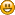BTW: was expecting it to update when I went into Machine Coord mode but it only computes Fixture offsets, Still a few things to work out.
But I am having FUN

Code: [Select]

function getHypot(axis1, axis2)
local hypot = math.sqrt(math.pow(axis1,2) + math.pow(axis2,2));
Hyp = hypot;
return Hyp;
end

function getAngle(axis1, axis2)
PI = math.pi;
local BaseAngle = radians * (180/PI)

if (axis1 == 0 and axis2 == 0) then
angle = 0;
end
if (axis2 < 0 and axis1 > 0) then
BaseAngle = -(90 + BaseAngle);
end
if (BaseAngle < 0) then
angle = (BaseAngle + 360);
elseif (BaseAngle > 0) then
angle = BaseAngle;
end
if (angle == 0 or angle == 90 or angle == 180 or angle == 270) then
if (axis1 > 0 or (axis1 == 0 and axis2 == 0)) then angle = 0;
elseif (axis1 < 0 and axis2 == 0) then angle = 180;
elseif (axis1 == 0 and axis2 > 0) then angle = 90;
elseif (axis1 == 0 and axis2 < 0) then angle = 270;
end
end
return angle
end

Code: [Select]
--PLC Script

local Xval = mc.mcAxisGetPos(0,0);
local Yval = mc.mcAxisGetPos(0,1);
local Zval = mc.mcAxisGetPos(0,2);

getAngle(Xval,Yval);
scr.SetProperty('droXY', 'Value', tostring(angle));
getAngle(Xval,Zval);
scr.SetProperty('droXZ', 'Value', tostring(angle));
getAngle(Yval,Zval);
scr.SetProperty('droYZ', 'Value', tostring(angle));

getHypot(Xval,Yval);
scr.SetProperty('droXYhyp', 'Value', tostring(Hyp));
getHypot(Xval,Zval);
scr.SetProperty('droXZhyp', 'Value', tostring(Hyp));
getHypot(Yval,Zval);
scr.SetProperty('droYZhyp', 'Value', tostring(Hyp));smurph••1,475
• "That there... that's an RV."Re: DRO Code differences
« Reply #14 on: April 28, 2014, 08:13:22 PM »
Is G68 not working?  Can you give an example?

SteveYa-Nvr-No•992Re: DRO Code differences
« Reply #15 on: April 28, 2014, 08:48:01 PM »
zero all axis's
MDI: G68 R45
sets coordinates to a 45 degree angle
DRO turn red in Mach3
if you jog in X you should see both X and Y move the same amount on a 45 degree angle
no luck at all in Mach4Brian Barker••3,854Re: DRO Code differences
« Reply #16 on: April 28, 2014, 08:50:24 PM »
Looks like it is working in my files

(2PanelNoOffset)
G68 r0
G00 G54
G90 G54
G61
S15000 M3
G4 P3
G0 X2 Y2 Z1
z.1
#10=1
M98 P1000 L20
G0 G90 Z1
G0 X6 Y2
z.1
G68 X6 Y2 R45
#10=1
M98 P1000 L20
X0.0 Y0.0
M5
G68 r0
M30

O1000
G91
G1 F10
Z-.1
X[#10]
Y[#10*-1]
X[#10*-1]
Y[#10]
#10=[#10+.1]
M99

Fixing problems one post at a timewww.newfangledsolutions.com
www.machsupport.comBrian Barker••3,854Re: DRO Code differences
« Reply #17 on: April 28, 2014, 08:51:36 PM »
DRO's don't change in Mach4...
Fixing problems one post at a timewww.newfangledsolutions.com
www.machsupport.comsmurph••1,475
• "That there... that's an RV."Re: DRO Code differences
« Reply #18 on: April 28, 2014, 08:51:51 PM »
Thanks!  I'll try it out.

It may be that the jog planners are not picking it up.  Seems to work fine in files.

SteveYa-Nvr-No•992Re: DRO Code differences
« Reply #19 on: April 28, 2014, 09:04:44 PM »
Your right if I do a
MDI: G1X1F10
it does move correctly
so it has to be in the jog routine.

I like the red DRO's